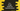# Singly linked list implementation in c++## What is a linked list :

A linked list is a data structure used to store items like a chain. Linked list is used to store unlimited amount of items. Each items are connected like a chain. Each item is called a Node. Each Node consists of data and link to the next node. It looks as like below :

Each rectangle is a node. The red part is the data and blue part is a pointer that **holds the address of the next node.

If we get access to the first node, we can iterate through each element of that linked list.

• The address of the first node is stored in a different pointer called Head.
• The pointer of the last node stores NULL

So, if we get the Head, we can traverse through the linked list, and also modify and delete any item of the list. In the above image, the green box is used to represent the head.

Singly linked list is an unidirectional linked list. We can traverse it only from one side. For example, the above images illustrates singly linked list. We also have one more type of linked list that can be traversed from both sides. But in this tutorial, we will talk only about singly linked list.

## Implementation of singly linked list in C++ :

In C++, we can implement singly linked list with the help of structure. We can create one structure that can act as a node like below :

``````struct Node
{
int value;
Node *next;
};``````

This structure can hold one int value and one pointer variable that can hold the address of another node. This will hold the address of the next node in the linked list.

### C++ implementation of singly linked list :

The below program implements singly linked list with the following two features :

1. Display the content of the list
2. Insert one new node at the head of the list i.e. at the position where we have the head.

For example, if we have one node with value 1 and we are adding one more node with value 2, the steps will be look as like below :

At first, the head is pointed to 1. Once we insert 2, it pushed 1 to right and 2 is added to the front with head pointed to 2.

Below is the complete C++ program :

``````#include <iostream>
using namespace std;

struct Node
{
int value;
Node *next;
};

{

public:
{
Node *node = new Node;
node->value = value;

{
node->next = NULL;
}
else
{
}
}

void displayList()
{
while (flag != NULL)
{
cout << flag->value << endl;
flag = flag->next;
}
}
};

int main()
{
int total, value;

cout << "Enter the total nodes :" << endl;
cin >> total;

for (int i = 0; i < total; i++)
{
cout << "Enter value for node " << i << " :" << endl;
cin >> value;
}

cout << "Printing the linked list :" << endl;
return 0;
}``````

### Sample Output :

``````Enter the total nodes :
4
Enter value for node 0 :
1
Enter value for node 1 :
3
Enter value for node 2 :
5
Enter value for node 3 :
7